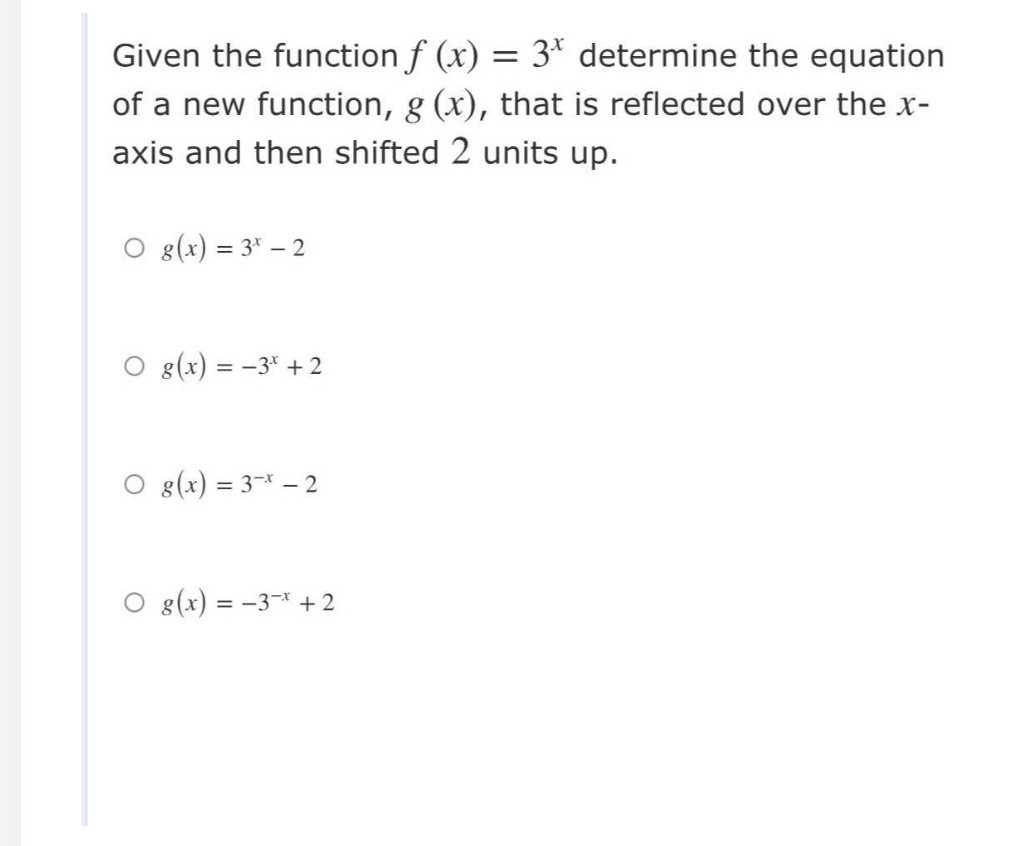### Still have math questions?

Algebra
QuestionGiven the function $$f ( x ) = 3 ^ { x }$$ determine the equation of a new function, $$g ( x ) ,$$ that is reflected over the $$x$$ - axis and then shifted $$2$$ units up.

$$\circ g ( x ) = 3 ^ { x } - 2$$

$$\circ g ( x ) = - 3 ^ { x } + 2$$

$$o g ( x ) = 3 ^ { - x } - 2$$

$$g ( x ) = - 3 ^ { - x } + 2$$

$$g ( x ) = - 3 ^ { x } + 2$$